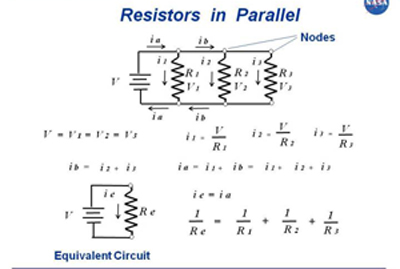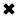# Resistances in Parallel#### Resistances in parallel are considered as conductances instead.

Resistances in Parallel

We can evaluate resistances in parallel by considering them as conductances instead. We express conductance in units called siemens, symbolized S. (The word "siemens" serves both in the singular and the plural sense). Some older physics or engineering documents use the mho ("ohm" spelled backwards) as the fundamental unit of conductance; the mho and the siemens represent exactly the same thing. When we connect conductances in parallel, their values add up, just as resistances add up in series. If we change all the ohmic values to siemens, we can add these figures up, and convert the final answer back to ohms.

Engineers use the uppercase, italic letter G to symbolize conductance as a parameter or mathematical variable. The conductance in siemens equals the reciprocal of resistance of the circuit equal to the sum in ohms, flowing through each resistor. We can express this fact using two formulas, assuming that neither R nor G ever equal zero:

G = 1/R

and

G = 1/G

Consider five resistors in parallel. Call the resistors R1 through R5, and call the total resistance R, as shown in Fig. 4-9. According to ohms law, suppose that the individual resistances have values of R1 = 100 ohms, R2 = 200 ohms, R3 = 300 ohms, R4 = 400 ohms, and R5 = 500 ohms. What's the total resistance R of this parallel combination? (Note that we do not italicize R when it means "resistor," but we do italicize R when it means "resistance.")Fig. 4-9. Five resistors R(1) through R(5), connected in parallel, produce a net resistance R.

When we connect sets of resistors to a source of voltage, each resistor draws some current. If we know the source voltage, we can figure out how much current the entire set demands by calculating the net resistance of the combination, and then considering the combination as a single resistor.

If the resistors that are connected in the network all have the same ohmic value, the power from the source divides up equally among them, whether we connect any number of resistors connected in series or in parallel. For example, if we have eight identical resistors in series with a battery, the network consumes a certain amount of power, each parallel resistor bearing 1/8 of the load. If we rearrange the circuit to connect the resistors in a parallel circuit with the same battery, the network as a whole dissipates a lot more power than it does when the resistors are in series circuit (no matter the voltage source), but each individual resistor handles 1/8 of the total power load, just as they do when they're in series. If the resistances in a network do not all have identical ohmic values, then some resistors dissipate more power than others.

##### Basic Electricity Handbook, Vol. 1• FULLY ILLUSTRATED
• GREAT FOR SCHOOL PROJECTS
• GREAT PRICE: \$5.99
• ...

This 100+ page e-book is a great guide for those who have a basic interest in the field of electricity. This well-illustrated e-book, coupled with some basic knowledge of electricity, will give you a broad theoretical background in this fundamental subject.

CONTENTS
• FUNDAMENTALS OF ELECTRICITY
• HOW DOES ELECTRICITY WORK?
• HOW IS ELECTRICITY GENERATED?
• WHERE DOES ELECTRICITY COME FROM?
• BASIC HOME WIRING
• ELECTRICITY FOR STUDENTS
• ENERGY SAVING TIPS

##### TRAINING EF COURSES

Content Community Connection
Top